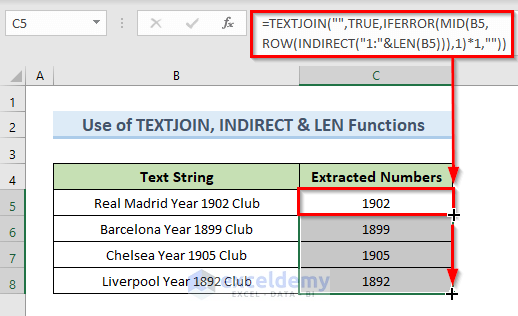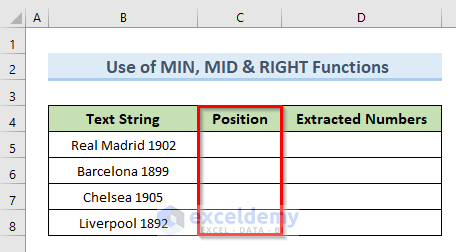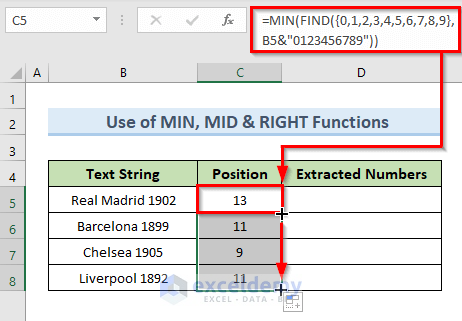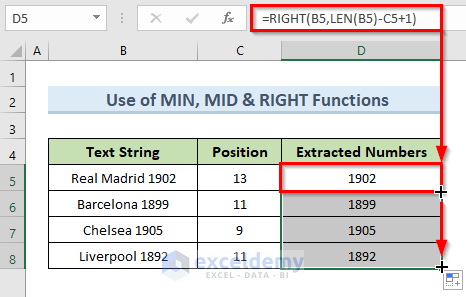# How to Extract Numbers after a Specific Text in Excel (2 Suitable Ways)

In this tutorial, we will demonstrate how to extract numbers after a specific text in Excel. In many instances, we need to extract a specific part of a string while working in Microsoft Excel. Excel doesn’t provide any dedicated function to do this. So, we have to use a combination of different excel functions to perform this action.

## 2 Suitable Ways to Extract Numbers after a Specific Text in Excel

In this article, we will discuss 3 suitable ways to extract numbers after a specific text in Excel. We can see an image of our dataset in the following screenshot. The dataset contains the names of four football clubs, as well as their founding years. The founding years part in the text string are in number format. So, we will extract that numeric part from the given dataset. For all the examples in this article, we will use the same dataset.### 1. Separate Numbers after a Particular Text with Excel Functions

In this part, we will separate numbers after a particular text when numbers can be anywhere in the text. To do this we will also use the combinations of different Excel functions.

#### 1.1 Insert TEXTJOIN, IFERROR, MID, ROW, INDIRECT & LEN Functions

In this method, we will use the combination of TEXTJOIN, IFERROR, MID, ROW, INDIRECT, and LEN functions to extract numbers after a specific text. To do this we will follow some simple steps.

STEPS:

• Firstly, select cell C5.
• Secondly copy the following formula in that cell:
`=TEXTJOIN("",TRUE,IFERROR(MID(B5,ROW(INDIRECT("1:"&LEN(B5))),1)*1,""))`
• Press Enter.
• So, in cell C5 the above command returns the number part from the string of cell B5.
• Thirdly, drag the Fill Handle tool from cell C5 to C8 to autofill the remaining dataset.
• Lastly, we get the result like the following image.

####🔎 How Does the Formula Work?

• INDIRECT(“1:”&LEN(B5)): The INDIRECT function takes the value of LEN(B5) as cell reference which is 26.
• ROW(INDIRECT(“1:”&LEN(B5))): The Row function uses the return value by the INDIRECT function as reference.
• MID(B5,ROW(INDIRECT(“1:”&LEN(B5))),1): In this part the MID function extracts the number parts from cell B5.
• IFERROR(MID(B5,ROW(INDIRECT(“1:”&LEN(B5))),1)*1,””): If the MID function finds a valid value the IFERROR function returns that otherwise it returns blank.
• TEXTJOIN(“”,TRUE,IFERROR(MID(B5,ROW(INDIRECT(“1:”&LEN(B5))),1)*1,””)): Here, the TEXTJOIN function returns the number part from the string in cell B5.

#### 1.2 Combine LOOKUP, MID, MIN & FIND Functions

In this method, we will use the LOOKUP, MID, MIN, and FIND functions to extract numbers after a specific text in Excel. We will find the numeric part after the text Year from our dataset. Follow the below steps to execute this method.

STEPS:

• To begin with, select cell C5.
• In addition, Type the below formula in th-at cell:
`=LOOKUP(10^6,1*MID(B5,MIN(FIND({0,1,2,3,4,5,6,7,8,9},B5&"0123456789",FIND("Year"," "&B5&" "))),{2,3,4,5,6}))`
• Press Enter.
• So, the above action returns the number part in cell C5 from the string of cell B5 after the text Year.
• Furthermore, drag the FIll Handle tool from cell C5 to C8 to autofill the dataset.
• In the end, we get results like the image below.🔎 How Does the Formula Work?

• FIND(“Year”,” “&B5&” “): This part finds the text value Year in cell B5.
• FIND({0,1,2,3,4,5,6,7,8,9},B5&”0123456789″,FIND(“Year”,” “&B5&” “)): This part finds the numeric values after the text Year in cell B5.
• MIN(FIND({0,1,2,3,4,5,6,7,8,9},B5&”0123456789″,FIND(“Year”,” “&B5&” “))): The MIN function returns the least position of the number characters.
• MID(B5,MIN(FIND({0,1,2,3,4,5,6,7,8,9},B5&”0123456789″,FIND(“Year”,” “&B5&” “))),{2,3,4,5,6}): The MID function extracts the number characters after the text Year from a string.
• LOOKUP(10^6,1*MID(B5,MIN(FIND({0,1,2,3,4,5,6,7,8,9},B5&”0123456789″,FIND(“Year”,” “&B5&” “))),{2,3,4,5,6})): This part will look for the specified conditions in cell B5. Then returns the number part after text Year from the string of cell B5.

#### 1.3 Apply MID & SEARCH Functions in Excel

Now, we will use the MID & SEARCH functions to extract numbers after specific text in excel. Just go through the below steps to perform this method.

STEPS:

• First, select cell C5.
• Next, write down the following formula in that cell:
`=MID(B5,SEARCH("Year ",B5)+4,SEARCH(" Club",B5)-SEARCH("Year ",B5)-4)`
• Hit Enter.
• So, in cell C5 we can see the value of the number part from the string of cell B5 after the text Year.
• Then, drag the FIll Handle tool from cell C5 to C8.
• Finally, we get the result like the following image.🔎 How Does the Formula Work?

• SEARCH(“Year “,B5): The SEARCH function returns the location of string Year inside the sting of cell B5.
• SEARCH(” Club”,B5)-SEARCH(“Year “,B5)-4: This part counts the characters between the strings Club and Year.
• MID(B5,SEARCH(“Year “,B5)+4,SEARCH(” Club”,B5)-SEARCH(“Year “,B5)-4): The MID function returns the number part between the strings Year and Club.

#### 1.4 Combination of VALUE, TRIM, MID, & FIND Functions

In this method, we will use the VALUE, TRIM, MID, and FIND functions to separate numbers after a particular text in Excel. To do this we will follow the below steps.

STEPS:

• In the beginning, select cell C5.
• Next, insert the following formula in that cell:
`=VALUE(TRIM(MID(B5,18,FIND("Club",B5)-19)))`
• Press Enter.
• Afterward, in cell C5 we get the numbers between the strings Year and Club of cell B5.• If you want to apply it to the cell B6 also insert the following formula in cell C6:
`=VALUE(TRIM(MID(B6,16,FIND("Club",B6)-17)))`
• Hit Enter.
• So, we get the result like the following image.🔎 How Does the Formula Work?

• FIND(“Club”,B6): Here the FIND function finds the position of string Club in cell B6.
• MID(B6,16,FIND(“Club”,B6)-17): The MID function selects the values from 16th character until the string Club.
• TRIM(MID(B6,16,FIND(“Club”,B6)-17))): TRIM function extracts the return portion defined by the previous part.
• VALUE(TRIM(MID(B6,16,FIND(“Club”,B6)-17))): The VALUE function in this part returns the numeric part of cell B6 in cell C6.

### 2. Use VBA Code to Extract Numbers after a Specific Text in Excel

We can also extract numbers after a specific text in excel with the use of VBA code. In this method, we will create a user-defined function. The user-defined function allows us to create customized functions. To apply VBA code in this method we will go through some simple steps.

STEPS:

• Firstly, go to the Developer tab.
• Secondly, select the option ‘Visual Basic’.• Thirdly, from the VBA code window select the Insert. From the menu, click on the option Module.• Next, in the blank VBA code window type the following code:
``````Function NumbersExtract(CellRef As String)
Dim StrLen As Integer
StrLen = Len(CellRef)
For i = 1 To StrLen
If (IsNumeric(Mid(CellRef, i, 1))) Then Result = Result & Mid(CellRef, i, 1)
Next i
NumbersExtract = Result
End Function``````
• Furthermore, click on the save button to save the code.
• So, the above code creates a user-defined function named NumberExtract to extract numbers after a specific text.• Then select cell C5. Type the user-defined function in cell C5 in the following way:
`=NumbersExtract(B5)`
• Press Enter.
• So, we can see the numbers of cell B5 after text Year in cell C5.
• After that, to auto-fill the data drag the Fill Handle tool from cell C5 to C8.
• Lastly, we get results like the following image.Read More: How to Separate Numbers from Text in Excel VBA (3 Methods)

## Extract Numbers If They Appear at End of Text Every Time in Excel

First and foremost, we will extract a number after a specific text if the numeric portion of the text always appears at the end of the text. To do this we will use a combination of different functions.

### 1. Combine MIN, FIND & RIGHT Functions to Extract Numbers

In the first method, we will use the MIN, FIND, and RIGHT functions to extract numbers after a specific text in Excel. We will insert an additional column to do this method. Let’s see the steps to perform this method.

STEPS:

• To begin with, insert a new column named Position.

####• In addition, select cell C5. Type the following formula in that cell:
`=MIN(FIND({0,1,2,3,4,5,6,7,8,9},B5&"0123456789"))`
• Press Enter. This action returns the starting position of the number in the string in cell C5.
• Furthermore, to autofill, the dataset, drag the Fill Handle tool from cell C5 to C8.

####🔎 How Does the Formula Work?

• FIND({0,1,2,3,4,5,6,7,8,9},B5&”0123456789″): Here the FIND function finds the numeric parts in cell B5.
• MIN(FIND({0,1,2,3,4,5,6,7,8,9},B5&”0123456789″)): The MIN function returns the first position of numbers in the text string of cell B5.
• Next, select cell D5. Insert the following formula in that cell:
`=RIGHT(B5,LEN(B5)-C5+1)`
• Press Enter.
• As a result, in cell D5 we get the value of the extracted numbers from a string in cell B5.
• After that, drag the Fill Handle tool from cell D5 to D8 to autofill the dataset.
• Lastly, we get the results like the following image.

####🔎 How Does the Formula Work?

• LEN(B5)-C5+1: The LEN function returns the length of cell B5. Subtract the value of cell C5 and add 1 with the value of LEN(B5).
• RIGHT(B5,LEN(B5)-C5+1): Here, the RIGHT function returns the strings after the position returned by the part LEN(B5)-C5+1.

Read More: How to Extract Specific Numbers from a Cell in Excel (11 Ways)

### 2. Split Numbers with Excel SUBSTITUTE, LEFT, MIN & FIND Functions

Now, we will use the SUBSTITUTE, LEFT, MIN, and FIND functions to pull out numbers after a particular text in excel. Let’s see the steps to this method.

STEPS:

• First, select cell C5.
• Next, type the following formula in that cell:
`=SUBSTITUTE(B5,LEFT(B5,MIN(IFERROR(FIND({0,1,2,3,4,5,6,7,8,9},B5),""))-1),"")`
• Then, hit Enter.
• So, in cell C5 the above action returns only the number part from the string of cell B5.
• After that, to autofill, the remaining part, drag the Fill Handle tool from cell C5 to C8.
• Finally, we can see the result in the image below. We get all the number parts from strings of cells (B5:B8) in cells (C5:C8).🔎 How Does the Formula Work?

• FIND({0,1,2,3,4,5,6,7,8,9},B5): The FIND function in this part finds the numeric parts in cell B5.
• IFERROR(FIND({0,1,2,3,4,5,6,7,8,9},B5),””): If the FIND function returns any error value the IFERROR function returns a blank value.
• MIN(IFERROR(FIND({0,1,2,3,4,5,6,7,8,9},B5),””))-1): The MIN function in this part returns the least position of number from the string in cell B5. In our example which is 12.
• LEFT(B5,MIN(IFERROR(FIND({0,1,2,3,4,5,6,7,8,9},B5),””))-1): Here, the LEFT function extracts 12 characters from the string in cell B5.
• SUBSTITUTE(B5,LEFT(B5,MIN(IFERROR(FIND({0,1,2,3,4,5,6,7,8,9},B5),””))-1),””): The SUBSTITUTE function substitutes the 12 characters with blank value and returns only the number parts.

Read More: How to Extract Multiple Numbers from String in Excel (6 Methods)

### 3. Use Flash Fill Feature to Extract Numbers If They Appear at End of Text in Excel

One interesting method to extract numbers after a specific text in excel is to use the Flash Fill feature. Flash Fill feature is only available in Microsoft Excel 365, Microsoft Excel 2013,  and later versions of Microsoft Excel 2013. We can use the method not only when the number part is at the end of the string but also for any positions of the number part in a string. Let’s see how we can do this easily.

STEPS:

• In the beginning, in cell C5 insert manually the value of the number part of the string in cell B5.• Next, start typing the number part of the next cell.
• If Excel detects a pattern, a preview of data to be auto-filled in the cells below will appear.• After that, to accept the suggestions press Enter.
• Finally, we can see our desired output in the following image.Read More: Extract Only Numbers from Excel Cell (6 Useful Methods)

## Conclusion

In conclusion, this tutorial is a complete guide on how to extract numbers after a specific text in Excel. Use the exercise worksheet that comes with this article to put your skills to the test. If you have any queries, please leave a comment below. Our team will try to respond as quickly as possible. Keep an eye out for more intriguing Microsoft Excel solutions in the future.

## Related Articles#### Mukesh Dipto

Hi there! I am Mukesh Dipto. I graduated with a bachelor's degree in engineering. Currently, I am working as a technical content writer in ExcelDemy. You will find all my articles on Microsoft Excel on this site. Outside of the workplace, my hobbies and interests include sports and meeting new people. I also enjoy sports. My favorite sports are football (to watch and play) and Badminton (play).

We will be happy to hear your thoughts5 Excel Hacks You Never Knew